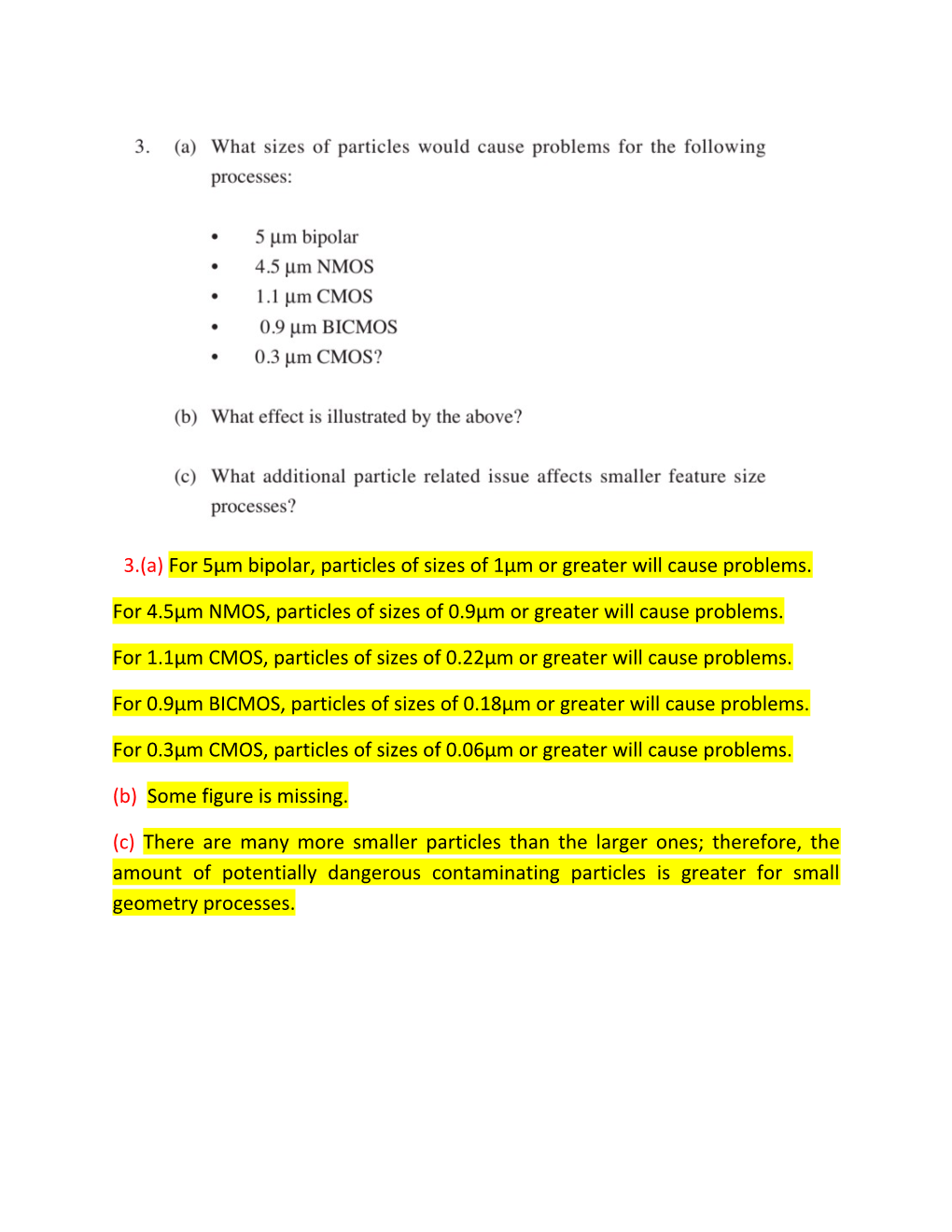# 3.(A)For 5Μm Bipolar, Particles of Sizes of 1Μm Or Greater Will Cause Problems3.(a)For 5µm bipolar, particles of sizes of 1µm or greater will cause problems.

For 4.5µm NMOS, particles of sizes of 0.9µm or greater will cause problems.

For 1.1µm CMOS, particles of sizes of 0.22µm or greater will cause problems.

For 0.9µm BICMOS, particles of sizes of 0.18µm or greater will cause problems.

For 0.3µm CMOS, particles of sizes of 0.06µm or greater will cause problems.

(b) Some figure is missing.

(c)There are many more smaller particles than the larger ones; therefore, the amount of potentially dangerous contaminating particles is greater for small geometry processes.(a) The maximum specification limit on the system is +100 particles of 0.5µm but we see that 350 particles of >0.5µm have been added. This is not acceptable as per the system requirements. Hence, the wafer as it is cannot be accepted for production. It will require cleaning of the wafer to bring particle count to the acceptable limit.

(b) Here the wafer is passing through process 1 only. For both the processes, the particle limit of size >0.5µm is 100; hence, limit on particles of size>0.5µm can be considered as 50 particles for process 1 only. Here we find that there are 334 particles of size >0.5µm. It cannot be accepted as per the system requirements. Something needs to be dome to reduce the number of particles to the acceptable limit before wafer can be considered for production.

(c) Here the wafer is passing through process 2 only. For both the processes, the particle limit of size >0.5µm is 100; hence, limit on particles of size>0.5µm can be considered as 50 particles for process 2 only. Here we find that there are 328 particles of size >0.5µm. It cannot be accepted as per the system requirements. Something needs to be dome to reduce the number of particles to the acceptable limit before wafer can be considered for production.

(d) The sources of particles are contamination, processes, clothes, skin, atmosphere etc.

The particles can be removed using wet wiping. Wet wiping is a more efficient way of cleaning hard surfaces and removes large amount of particles. Alcohol wipe downs have the added advantage of being anti-bacterial, and they evaporate to have very little residual. When cleaning, vacuuming could be used first to pick up the larger particles but wet wiping must then be used to pick up the smaller particles.

5.(a) Number of chips N =

Here N =?, A = 0.5cm2 = 50 mm2 as 1 cm2 = 100mm2. D = 300

Putting these values, we get

Number of defects = 2 per wafer per lithographic process

There are 6 lithographic processes.

Hence, number of defects = 2*6 = 12

Hence, yield = [(1347-12)/1347]*100= 99.109%

(b) Number of chips N =

Here N =?, A = 1cm2 = 100 mm2 as 1 cm2 = 100mm2. D = 300

Putting these values, we get

Number of defects = 2 per wafer per lithographic process

There are 6 lithographic processes.

Hence, number of defects = 2*6 = 12

Hence, yield = [(660-12)/660]*100= 98.18%

(c) Number of chips N =

Here N =?, A = 2cm2 = 200 mm2 as 1 cm2 = 100mm2. D = 300

Putting these values, we get

Number of defects = 2 per wafer per lithographic process

There are 6 lithographic processes.

Hence, number of defects = 2*6 = 12

Hence, yield = [(320-12)/320]*100= 96.25%

(d) Number of chips N =

Here N =?, A = 4cm2 = 400 mm2 as 1 cm2 = 100mm2. D = 300

Putting these values, we get

Number of defects = 2 per wafer per lithographic process

There are 6 lithographic processes.

Hence, number of defects = 2*6 = 12

Hence, yield = [(153-12)/153]*100= 92.156%

As chip size increases, yield decreases.